Courses

# Refrigerant Compressors - MCQ Test 2

## 30 Questions MCQ Test RRB JE for Mechanical Engineering | Refrigerant Compressors - MCQ Test 2

Description
This mock test of Refrigerant Compressors - MCQ Test 2 for Mechanical Engineering helps you for every Mechanical Engineering entrance exam. This contains 30 Multiple Choice Questions for Mechanical Engineering Refrigerant Compressors - MCQ Test 2 (mcq) to study with solutions a complete question bank. The solved questions answers in this Refrigerant Compressors - MCQ Test 2 quiz give you a good mix of easy questions and tough questions. Mechanical Engineering students definitely take this Refrigerant Compressors - MCQ Test 2 exercise for a better result in the exam. You can find other Refrigerant Compressors - MCQ Test 2 extra questions, long questions & short questions for Mechanical Engineering on EduRev as well by searching above.
QUESTION: 1

### 0.70 kg/s of air enters with a specific enthalpy of 290 kJ and leaves it with 450 kJ of specific enthalpy. Velocities at inlet and exit are 6 m/s and 2 m/s respectively. Assuming adiabatic process, what is power input to the compressor?

Solution:

Power input to compressor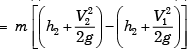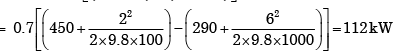QUESTION: 2

Solution:
QUESTION: 3

### The air with enthalpy of 100kJ/kg is compressed by an air compressor to a pressure and temperature at which its enthalpy becomes 200kJ/kg. The loss of heat is 40 kJ/kg from the compressor as· the air passes through it.  Neglecting kinetic and potential energies, the power required for an air mass flow of 0.5kg/s is:

Solution:
QUESTION: 4

The discharge pressure of the compressor in the refrigeration system goes up due to the

Solution:
QUESTION: 5

Which one of the following statements is true?

Solution:
QUESTION: 6

Two-stage compressors takes in air at 1.1 bar and discharges at 20 bar. For maximum efficiency, the intermediate pressure is:

Solution:

We know that for minimum compressor work pressure ratio of both stages must be same so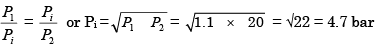QUESTION: 7

A 3-stage reciprocating compressor has suction pressure of 1 bar and delivery pressure of 27 bar. For minimum work of compression, the delivery pressure of 1st stage is:

Solution:

For minimum work of compression in 3 stage compressor the delivery pressure of 1st stage is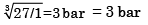QUESTION: 8

The heat rejection by a reciprocating air compressor during the reversible compression process AB, shown in the following temperatureentropy diagram, is represented by the area: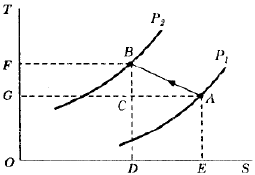Solution:

Heat rejection during AB is given by area below it on entropy axis, i.e. ABDE. DES

QUESTION: 9

Phenomenon of choking in compressor means

Solution:

Phenomenon of choking in compressor means fixed mass flow rate regardless of pressure ratio.

QUESTION: 10

Assertion (A ): T he isothermal efficiency of a reciprocating compressor becomes 100% if perfect cooling of the fluid during compression is attained.

Reason (R): Wor k do ne in a reciprocating compressor is less if the process of compression is isothermal rather than polytropic.

Solution:

Both assertion and reason are correct and R provides correct explanation for A.

QUESTION: 11

The suction pressure is 1 bar and delivery pressure is 125 bar. What is the ideal intermediate pressure at the end of first stage for a 3-stage air compressor?

Solution:
QUESTION: 12

When the discharge pressure is too high in a refrigeration system, high pressure control is installed to:

Solution:
QUESTION: 13

Which one of the following statements is true?

Solution:
QUESTION: 14

For a multistage compressor, the polytropic efficiency is:

Solution:

For multistage compressor, the polytropic efficiency is the efficiency of all stages combined together.

QUESTION: 15

The usual assumption in elementary compressor cascade theory is that

Solution:

The usual assumption in elementary compressor cascade theory is that axial velocity thr6ugh the cascade does not change.

QUESTION: 16

In a reciprocating air compressor the compression works per kg of air.

Solution:

Compression work per kg. of air increases as clearance volume increases.

QUESTION: 17

Consider the following statements:

1. Reciprocating compressors are best suited for high pressure and low volume capacity.

2. The effect of clearance volume on power consumption is negligible for the same volume of discharge

3. While the compressor is idling, the delivery valve is kept open by the control circuit.

4. Inter-cooling of air between the stages of compression helps to minimize losses.

Of these statements:

Solution:
QUESTION: 18

In a two-stage reciprocating air compressor, the suction and delivery pressures are 1 and 4 bar respectively. For maximum efficiency, the intercooler pressure is

Solution:
QUESTION: 19

The optimum intermediate pressure Pi for a gas turbine plant operating between pressure limits P1 and P2 with perfect inter cooling between the two stages of compression (with identical isentropic efficiency is given by:

Solution:

We know that for minimum compressor work pressure ratio of both stage must be same so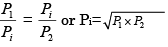QUESTION: 20

3-stage reciprocating compressors have suction pressure of 1 bar and delivery pressure of 27 bar. For minimum work of compression, the delivery pressure of first stage is:

Solution:

We know that for minimum compressor work pressure ratio of 3-stage must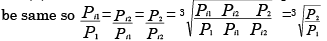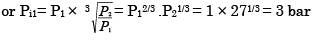QUESTION: 21

Four-stage compressor with perfect inter-cooling between stages compresses air from 1 bar to 16 bar. The optimum pressure in the last intercooler will be:

Solution:

We know that for minimum compressor work pressure ratio of 4-stage must be same so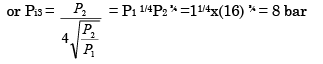QUESTION: 22

Which of the following statements is not correct for the volumetric efficiency of a reciprocating air compressor?

Solution: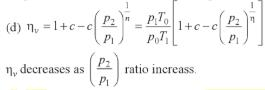QUESTION: 23

Which of the following statements are correct for multi staging in a reciprocating air compressor?

1.It decreases the volumetric efficiency.

2.The work done can be reduced.

3. small high-pressure cylinder is required.

4.The size of flywheel is reduced.

Select the correct answer using the codes given below

Solution:

1 is false, it increases the volumetric efficiency.

QUESTION: 24

Consider the following statements

The volumetric efficiency of a reciprocating compressor can be enhanced by:

1. Heating the intake air

2. Decreasing the clearance volume

3. Cooling the intake air

Which of these statements is/are correct?

Solution:
QUESTION: 25

The ratio of the clearance volume to the displacement volume of a R12 reciprocating compressor is 0.05. Specific volume at inlet and outlet of compressor are 0.04 and 0.02 m3/kg respectively. Volumetric efficiency of the compressor is:

Solution:
QUESTION: 26

Consider the following statements:The volumetric efficiency of a compressor depends upon

1. Clearance volume

2. Pressure ratio

3. Index of expansion

Of these correct statements are:

Solution:

The volumetric efficiency of a compressor depends upon

1. Clearance volume

2. Pressure ratio

3. Index of expansion

QUESTION: 27

A gas engine has a swept volume of 300 cc and clearance volume of 25 cc. Its volumetric efficiency is 0.88 and mechanical efficiency is 0.90. What is the volume of the mixture taken in per stroke?

Solution: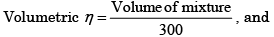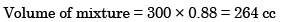QUESTION: 28

Which of the following techniques are employed for control of reciprocating compressors?

1. Throttle control

2. Clearance control

3. Blowing air to waste

Select the correct answer using the code given below:

Solution:
QUESTION: 29

What does application of centrifugalair compressors lead to

Solution:
QUESTION: 30

In centrifugal compressor terminology, vane less space refers to the space between

Solution: